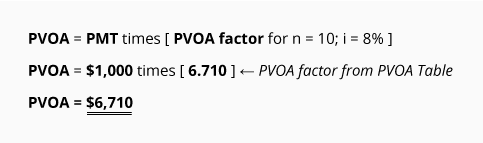# Present Value Formulas, Tables and Calculators

The easiest and most accurate way to calculate the present value of any future amounts (single amount, varying amounts, annuities) is to use an electronic financial calculator or computer software. Some electronic financial calculators are now available for less than \$35.

IMPORTANT! Understand that each financial calculator operates differently and may operate in a manner that is different from our Explanation. Be certain to read and thoroughly understand the directions before operating any calculator or computer software. One incorrect assumption, one incorrect input, or the use of a button that works differently from what you assumed, will mean an incorrect answer—and that could have a significant financial consequence!

If you don't have access to an electronic financial calculator or software, an easy way to calculate present value amounts is to use present value tables (PV tables). PV tables cannot provide the same level of accuracy as financial calculators or computer software because they use factors that are rounded off to fewer decimal places. In addition, they usually contain a limited number of choices for interest rates and time periods. Despite this, present value tables remain popular in academic settings because they are easy to incorporate into a textbook. Because of their widespread use, we will use present value tables for solving our examples.

Behind every table, calculator, and piece of software, are the mathematical formulas needed to compute present value amounts, interest rates, number of periods, payment amounts, and other future value amounts. We will use present value tables throughout our explanation.

Except for minor differences due to rounding, answers to the exercises below will be the same whether they are computed using a financial calculator, computer software, PV tables, or formulas.

### Using the PVOA Table

If you don't have access to an electronic financial calculator or software, an easy way to calculate present value amounts is to use present value tables. You can view a present value of an ordinary annuity table by clicking PVOA Table.

The first column (n) refers to the number of recurring identical payments (or periods) in an annuity. The other columns contain the factors for the interest rate (i) specified in the column heading. The point where a particular interest rate (i) intersects a particular number of payments (n) is the annuity's PVOA factor. When you multiply this factor by the annuity's recurring payment amount, the result is the present value of the annuity.

If you know an annuity is discounted at 8% per period and there are 10 periods, look on the PVOA Table for the intersection of i = 8% and n = 10. You will find the factor 6.710. Once you know the factor, simply multiply it by the amount of the recurring payment; the result is the present value of the ordinary annuity.

Let's demonstrate the use of the PVOA factors. If an ordinary annuity consists of 10 payments of \$1,000 each and the interest rate for discounting is 8%, the present value of the ordinary annuity is:If you are familiar with our topic Present Value of a Single Amount, you will notice that the result here is the same as if we had discounted each of the \$1,000 payments by using a PV of 1 Table and then summed the ten present value amounts. Rather than doing those ten calculations, however, the PVOA Table has combined for us the 10 factors from the PV of 1 Table—this allows us to compute the present value with just one multiplication. Note that this is possible only when each of the ten payments is identical in amount. (If the ten payments varied in amount, we could not use the annuity table; we would have to calculate the present value of a single amount for each of the ten payments and then sum the ten present value amounts.)